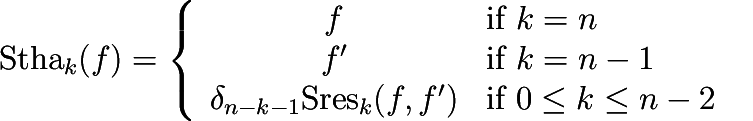CGAL 4.7 - Polynomial
PolynomialTraits_d::SturmHabichtSequence Concept Reference

Definition

Note: This functor is optional!

Computes the Sturm-Habicht sequence (aka the signed subresultant sequence) of a polynomial $$f$$ of type PolynomialTraits_d::Polynomial_d with respect to a certain variable $$x_i$$. The Sturm-Habicht sequence is similar to the polynomial subresultant sequence of $$f$$ and its derivative $$f':=\frac{\partial f}{\partial x_i}$$ with respect to $$x_i$$. The implementation is based on the following definition:

Let $$n:=\deg f$$ and $$\delta_k:=(-1)^{k(k+1)/2}$$. For $$k\in\{0,\ldots,n\}$$, the $$k$$-th Sturm-Habicht polynomial of $$f$$ is defined as:where $$\mathrm{Sres}_k(f,f')$$ is defined as in the concept PolynomialTraits_d::PolynomialSubresultants.

The result is written in an output range, starting with the $$0$$-th Sturm-Habicht polynomial (which is equal to the discriminant of $$f$$ up to a multiple of the leading coefficient).

Refines:
Polynomial_d
PolynomialTraits_d
PolynomialTraits_d::Resultant
PolynomialTraits_d::PrincipalSturmHabichtSequence
PolynomialTraits_d::SturmHabichtSequenceWithCofactors
PolynomialTraits_d::PolynomialSubresultants

Operations

template<typename OutputIterator >
OutputIterator operator() (Polynomial_d f, OutputIterator out)
computes the Sturm-Habicht sequence of $$f$$, with respect to the outermost variable. More...

template<typename OutputIterator >
OutputIterator operator() (Polynomial_d f, OutputIterator out, int i)
computes the Sturm-Habicht sequence of $$f$$ with respect to the variable $$x_i$$.

Member Function Documentation

template<typename OutputIterator >
 OutputIterator PolynomialTraits_d::SturmHabichtSequence::operator() ( Polynomial_d f, OutputIterator out )

computes the Sturm-Habicht sequence of $$f$$, with respect to the outermost variable.

Each element is of type PolynomialTraits_d::Polynomial_d.